Next: Error Estimation Up: Estimation of Physical Parameters Previous: Spikes' Remotion   Contents

## Total Counts

The total counts of a GRB, for the GRBM and AC energy bands, are derived from the background subtracted light curves. The total counts are simply calculated by summing the background subtracted counts of all the bins that are inside the GRB interval, whose start and end times have to be chosen by human intervention after visual inspection of the overall set of 8 light curves. Eq.expresses this procedure mathematically.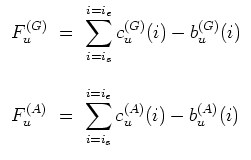(41)

In eq.,and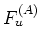are the total counts (or fluences, expressed in counts) for the GRBM and AC energy bands in the unit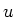, respectively; the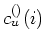and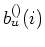are the measured and the background counts at the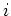-th bin, respectively;and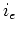are the bin numbers of the start and of the end times, respectively, as they are chosen by visual inspection.

Of course, this point is one the most delicate, since there could be some bursts, whose start and/or end times could be significantly different, depending on whether one considers a given structure in the light curves as true GRB signal or mere background variations.

SubsectionsNext: Error Estimation Up: Estimation of Physical Parameters Previous: Spikes' Remotion   Contents
Cristiano Guidorzi 2003-07-31